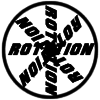#### You may also like### 8 Methods for Three by One

This problem in geometry has been solved in no less than EIGHT ways by a pair of students. How would you solve it? How many of their solutions can you follow? How are they the same or different? Which do you like best?### Rots and Refs

Follow hints using a little coordinate geometry, plane geometry and trig to see how matrices are used to work on transformations of the plane.### Reflect Again

Follow hints to investigate the matrix which gives a reflection of the plane in the line y=tanx. Show that the combination of two reflections in intersecting lines is a rotation.

# Transformations for 10

##### Age 16 to 18 Challenge Level:

The operation of mutiplying a vector by a constant matrix can by thought of as transforming a point in space onto another point in space. These transformations can have very clear, intuitive properties and we can often think of them from either a geometrical perspective or an algebraic perspective.

Below are ten questions about the properties of such transformations in three dimensions for you to think about.

As you think about the questions, can you draw relevant diagrams and construct relevant algebraic examples? In each case, is there a definitive answer, or does it depend on various factors? You may intuitively feel the answers to some of these questions; in these cases can you prove your intuition correct?

1. What does a matrix do to the zero vector ${\bf 0}$?
2. What does a matrix do to a line/plane through the origin?
3. What does a matrix do to a line/plane not through the origin?
4. Which lines can you transform onto the x-axis using matrix multiplication?
5. Which planes can you transform onto the xy-plane using matrix multiplication?
6. Can you think of a matrix which transforms a plane to a line?
7. Can you think of a matrix which transforms a line to a plane?
8. How many matrices transform the cube $(\pm 1, \pm 1, \pm 1)$ to another cube?
9. Can you find a matrix which transforms a square to a triangle in 2D?
10. Can you think of a matrix which shifts all points from ${\bf x}$ to ${\bf x+ (1,0,0)}$?